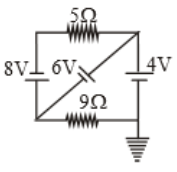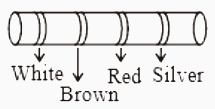The current through the 5$\mathrm{\Omega }$ resistor is(1) 3.2A

(2) 2.8A

(3) 0.8A

(4) 0.2A

Concept Questions :-

Kirchoff's voltage law
High Yielding Test Series + Question Bank - NEET 2020

Difficulty Level:

The current in a wire varies with time according to the relation i= (3+2t)A. The amount of charge passing a cross section of the wire in the time interval t=0 to t=4.0 sec would be (where t is time in seconds)

1.  28 C

2.  30.5 C

3.  8 C

4.  82 C

Concept Questions :-

Current and current density
High Yielding Test Series + Question Bank - NEET 2020

Difficulty Level:

In the figure, a carbon resistor has bands of different colours on its body as shown. The value of the resistance is1.  2.2 $\mathrm{K\Omega }$

2.  3.3 $\mathrm{K\Omega }$

3.  5.6 $\mathrm{K\Omega }$

4.  9.1 $\mathrm{K\Omega }$

Concept Questions :-

Colour coding of resistors
High Yielding Test Series + Question Bank - NEET 2020

Difficulty Level:

The resistivity of iron is 1 × 10–7 ohm m. The resistance of iron wire of particular length and thickness is 1 ohm. If the length and the diameter of wire both are doubled, then the resistivity in ohm m will be :

(1) 1 × 10–7

(2) 2 × 10–7

(3) 4 × 10–7

(4) 8 × 10–7

Concept Questions :-

Derivation of Ohm's law
High Yielding Test Series + Question Bank - NEET 2020

Difficulty Level:

The temperature coefficient of resistance for a wire is 0.00125/°C. At 300K its resistance is 1 ohm. The temperature at which the resistance becomes 2 ohm is

(1) 1154 K

(2) 1100 K

(3) 1400 K

(4) 1127 K

Concept Questions :-

Derivation of Ohm's law
High Yielding Test Series + Question Bank - NEET 2020

Difficulty Level:

When the length and area of cross-section both are doubled, then its resistance :

(1) Will become half

(2) Will be doubled

(3) Will remain the same

(4) Will become four times

Concept Questions :-

Derivation of Ohm's law
High Yielding Test Series + Question Bank - NEET 2020

Difficulty Level:

The resistivity of a wire :

(1) Increases with the length of the wire

(2) Decreases with the area of cross-section

(3) Decreases with the length and increases with the cross-section of the wire

(4) None of the above statement is correct

Concept Questions :-

Derivation of Ohm's law
High Yielding Test Series + Question Bank - NEET 2020

Difficulty Level:

Drift velocity vd varies with the intensity of electric field as per the relation

(1) ${v}_{d}\propto E$

(2) ${v}_{d}\propto \frac{1}{E}$

(3) vd = constant

(4) ${v}_{d}\propto {E}^{2}$

Concept Questions :-

Current and current density
High Yielding Test Series + Question Bank - NEET 2020

Difficulty Level:

In a conductor 4 coulombs of charge flows for 2 seconds. The value of electric current will be :

(1) 4 volts

(2) 4 amperes

(3) 2 amperes

(4) 2 volts

Concept Questions :-

Current and current density
High Yielding Test Series + Question Bank - NEET 2020

Difficulty Level:

The specific resistance of a wire is ρ, its volume is 3 m3 and its resistance is 3 ohms, then its length will be

(1) $\sqrt{\frac{1}{\rho }}$

(2) $\frac{3}{\sqrt{\rho }}$

(3) $\frac{1}{\rho }\sqrt{3}$

(4) $\rho \sqrt{\frac{1}{3}}$

Concept Questions :-

Derivation of Ohm's law